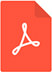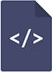# Unique energy levels and shifts in the heat drop of the universe from the Planck temperature to the present day based on a combined Einstein gravitational field equationPDF Full Text

# Abstract

In the general relativity, it is possible to determine the size and age of the universe (13.73 billion years) by means of a newfound Einstein gravitational field equation. Multiplied the mass of the Earth by the ratio of the complete angle (2?π) and of the deviation angle of a light beam grazing the surface of the Earth (α/2?π), towards the macro and microcosm three times, both of the present total (5.4?1053kg) and of the initial mass of the universe (6.49?10-5kg) can be calculated. Testing the classic formula of Planck temperature (T=M?c2/k), in the case of the initial mass of the cosmos / which is (π?) 950.08 times the Planck mass /, the initial temperature of the universe could be determined as well. Using the ratio of the angles for one to three times, the temperature would gradually decrease and finally reach the surface temperature of the lightened ancient cosmos. Due to the Planck temperature restoration the derivative primordial temperatures / 2·π?(3.13?1022K, 6.95?1012K and 1541.1 K) / are also shifted by the factor of (π?) 950.08 to the lower values, finally cooling the universe to the present amount (2·π?0.516 K). Around these stages of the developing cosmos, forward in time after the quark-gluon plasma state of matter all of the elementary particles and of the entire spectrum of electromagnetic radiation could be identified. By dividing both the present total and the initial mass of the universe by the factor, the visible matter of the cosmos (1.79?1050kg) and the Planck mass remain.

# Keywords

energy levels, gravitational field equation, heat drop, universe, milky way galaxy